# RandomExhaustive Search 2 Generate and Test Generate a

• Slides: 11
Download presentationRandom/Exhaustive Search 2. Generate and Test Generate a candidate solution and test to see if it solves the problem Repeat l Information used by this algorithm l 1. • Computer Science You know when you have found the solution Genetic Algorithms Slide 1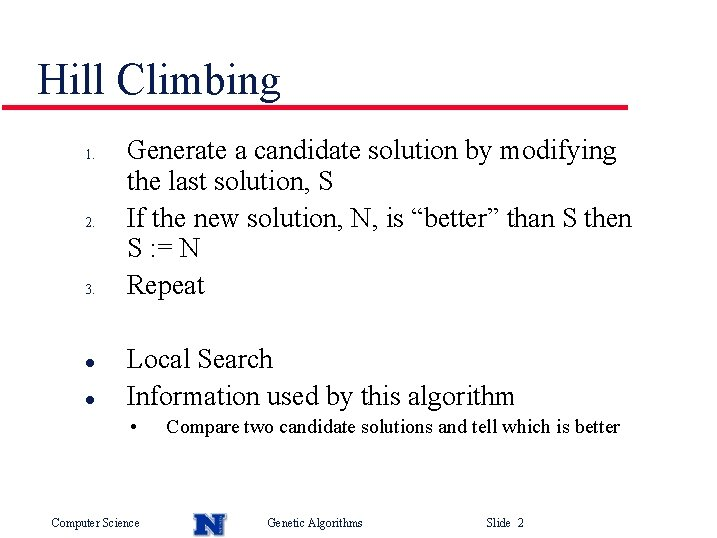Hill Climbing 1. 2. 3. l l Generate a candidate solution by modifying the last solution, S If the new solution, N, is “better” than S then S : = N Repeat Local Search Information used by this algorithm • Computer Science Compare two candidate solutions and tell which is better Genetic Algorithms Slide 2Population of Hill Climbers l Randomly generate initial population of hill climbers (Randomly generate initial candidate solutions) Do hill climbing in parallel After time t, choose best solution in population l Information used by this algorithm l l • Computer Science Same as hill climbing Genetic Algorithms Slide 3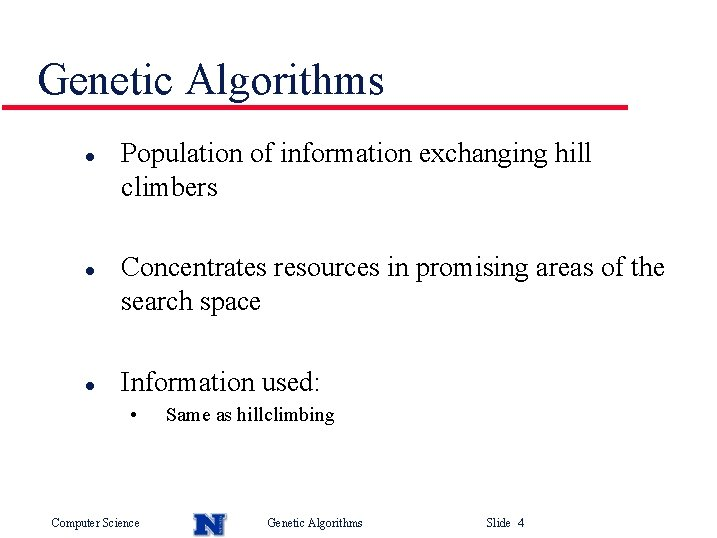Genetic Algorithms l l l Population of information exchanging hill climbers Concentrates resources in promising areas of the search space Information used: • Computer Science Same as hillclimbing Genetic Algorithms Slide 4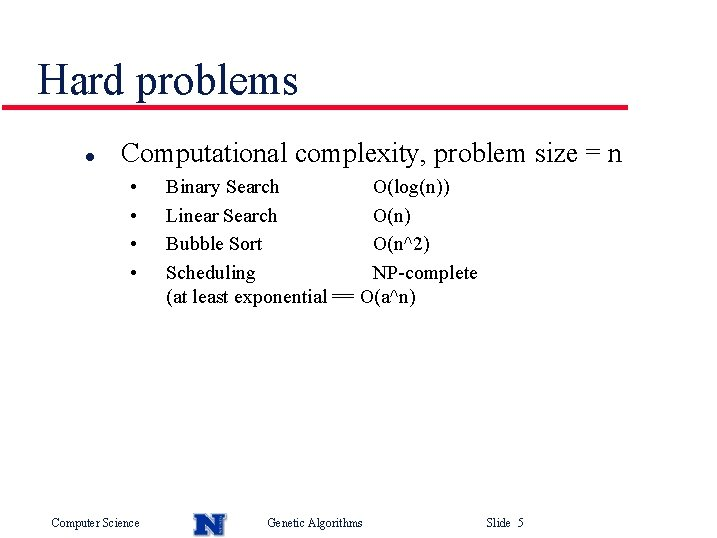Hard problems l Computational complexity, problem size = n • • Computer Science Binary Search O(log(n)) Linear Search O(n) Bubble Sort O(n^2) Scheduling NP-complete (at least exponential == O(a^n) Genetic Algorithms Slide 5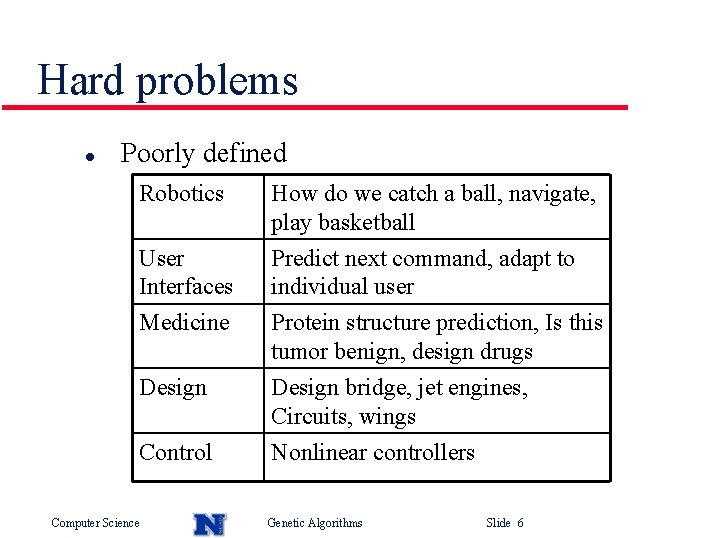Hard problems l Poorly defined Robotics How do we catch a ball, navigate, play basketball User Interfaces Predict next command, adapt to individual user Medicine Protein structure prediction, Is this tumor benign, design drugs Design bridge, jet engines, Circuits, wings Design Control Computer Science Nonlinear controllers Genetic Algorithms Slide 6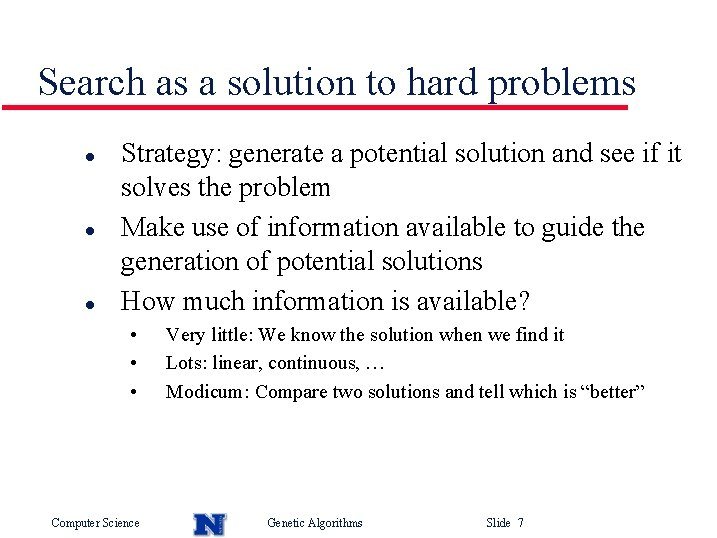Search as a solution to hard problems l l l Strategy: generate a potential solution and see if it solves the problem Make use of information available to guide the generation of potential solutions How much information is available? • • • Computer Science Very little: We know the solution when we find it Lots: linear, continuous, … Modicum: Compare two solutions and tell which is “better” Genetic Algorithms Slide 7Search tradeoff l l l Very little information for search implies we have no algorithm other than RES. We have to explore the space thoroughly since there is no other information to exploit Lots of information (linear, continuous, …) means that we can exploit this information to arrive directly at a solution, without any exploration Modicum of information (compare two solutions) implies that we need to use this information to tradeoff exploration of the search space versus exploiting the information to concentrate search in promising areas Computer Science Genetic Algorithms Slide 8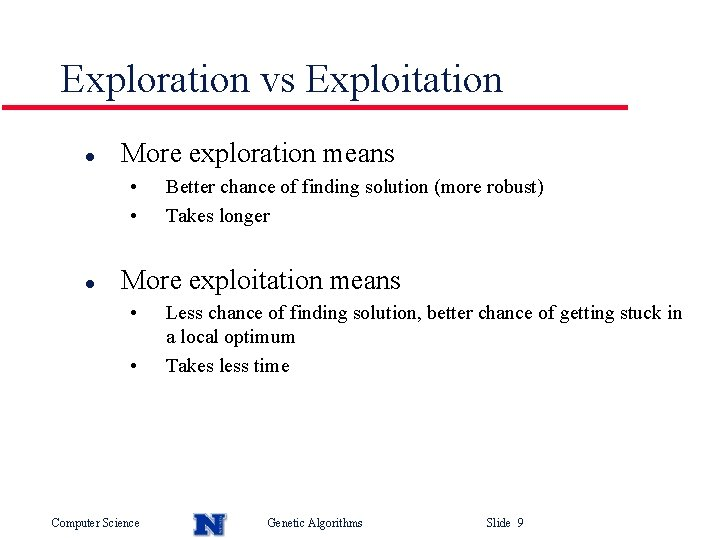Exploration vs Exploitation l More exploration means • • l Better chance of finding solution (more robust) Takes longer More exploitation means • • Computer Science Less chance of finding solution, better chance of getting stuck in a local optimum Takes less time Genetic Algorithms Slide 9Choosing a search algorithm l l l The amount of information available about a problem influences our choice of search algorithm and how we tune this algorithm How does a search algorithm balance exploration of a search space against exploitation of (possibly misleading) information about the search space? What assumptions is the algorithm making? Computer Science Genetic Algorithms Slide 10Genetic Algorithm l l Generate pop(0) Evaluate pop(0) T=0 While (not converged) do • • l Select pop(T+1) from pop(T) Recombine pop(T+1) Evaluate pop(T+1) T=T+1 Done Computer Science Genetic Algorithms Slide 11